# Matplotlib Line Plot – Complete Tutorial for Beginners

## Introduction

In this article, we will go through the tutorial for the matplotlib line plot. Line plots are the basic visualization that is used very much in machine learning, data science projects. In the tutorial, we will learn how line plots can be presented with different appearances with many useful examples

### Importing Matplotlib Library

Before beginning with this matplotlib bar plot tutorial, we’ll have to import Matplotlib and other required libraries for our examples.

In :
import matplotlib.pyplot as plt
import numpy as np
import pandas as pd


## Matplotlib Line Plot

A line plot or line chart is a graph that represents the frequency of data points along a number line. It is recommended to use a line plot with a small dataset of values. Line Plot gives the advantage of visualizing the data in a quick and efficient manner.

### Example 1: Simple Matplotlib Line Plot

Line Plot is the simplest of all the plots using which we can easily plot a line function.

Here to generate data, we have used the linspace function for the x-axis and log function for the y-axis.

The functions xlim and ylim are used for setting the range of x-axis and y-axis.

In :
x=np.linspace(1,200,75)
y=np.log(x)
plt.plot(x,y)
plt.xlabel('x')
plt.ylabel('logx')
plt.title('Simple Line Plot')
plt.xlim(0,100)
plt.ylim(0,5)

Output: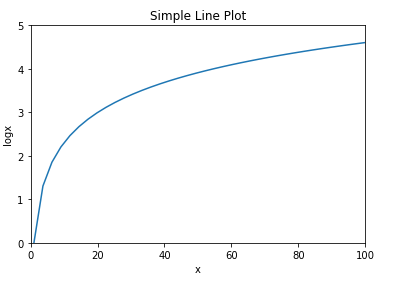### Example 2: Multiple Lines Plot

This 2nd example tells us how we can plot multiple lines. First, data is generated using linspace function. For plotting multiple lines, we use plot function multiple times.

After setting the range of x-axis and y-axis, we call the show function for producing the final output.

In :
# Multiple lines in same plot
x=np.linspace(1,40,200)

# Plot
plt.plot(x, np.sin(x))
plt.plot(x,np.log(x))
plt.plot(x,np.cos(-x))

# Decorate
plt.xlabel('x')
plt.title('Multiple Lines Plot')
plt.xlim(1,10)
plt.ylim(-1.0 , 2.5)
plt.show()

Output: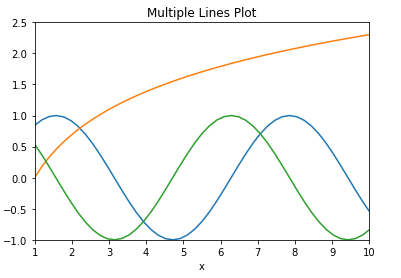### Example 3: Displaying different lines in different styles

As we did in previous examples, we have to generate random data using arange function. For different line styles, we pass the linestyle parameter in plot function.

In :
# Change line style
x=np.arange(0,50,0.5)

# Plot
plt.plot(x, np.sin(x), linestyle='dashed')
plt.plot(x, np.sin(x+0.5), linestyle='dashdot');
plt.plot(x, np.cos(x+1.0),linestyle = '--')
plt.plot(x, np.cos(x),linestyle=':')

# Decorate
plt.xlim(0,20)
plt.ylim(-1.00, 1.00)

Output: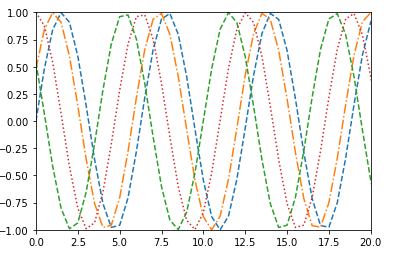### Example 4: Line Plot with Markers

In this example, we will look to incorporate markers in our line plot. Once we decide the number of lines to be plotted and set the figure size. To plot multiple lines, we call the plot function multiple times.

After setting the labels, we can pass the legend function for setting legends in an appropriate position.

In :
builds = np.array([1, 2, 3, 4])
y_stack = np.row_stack(([8, 9, 5, 4], [5, 2, 9, 1], [20, 10, 15, 1], [5, 10, 15, 20]))

fig = plt.figure(figsize=(11,8))

ax1.plot(builds, y_stack[0,:], label='Component 1', color='c', marker='*')
ax1.plot(builds, y_stack[1,:], label='Component 2', color='g', marker='*')
ax1.plot(builds, y_stack[2,:], label='Component 3', color='r', marker='*')
ax1.plot(builds, y_stack[3,:], label='Component 4', color='b', marker='*')

plt.xticks(builds)
plt.xlabel('Builds')

handles, labels = ax1.get_legend_handles_labels()
lgd = ax1.legend(handles, labels, loc='upper center', bbox_to_anchor=(1.15,1))
ax1.grid('on')

plt.show()

Output: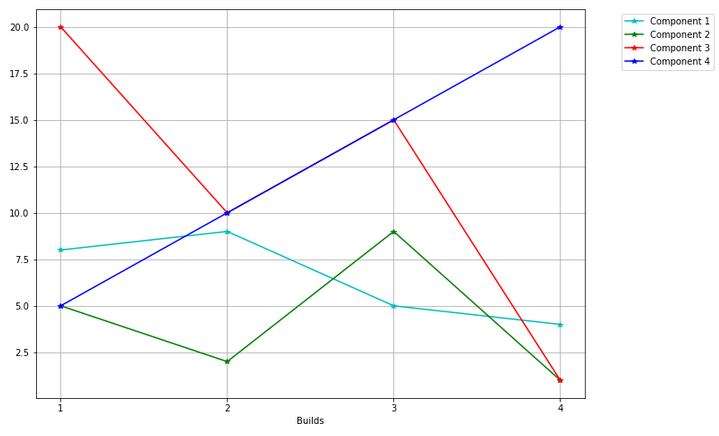### Example 5: Stacked Line Plot

This kind of line plot helps in comparing multiple line plots. With the help of subplot() function, we can plot our line plot along with different features. To plot stacked lines, we call the plot function.

With the help of colormap, we assign different colors to the lines used in stacked line plot.

In :
fnx = lambda : np.random.randint(4, 10, 10)
y = np.row_stack((fnx(), fnx(), fnx(), fnx()))

x = np.arange(10)
y_stack = np.cumsum(y, axis=0)

fig = plt.figure(figsize=(11,8))

ax1.plot(x, y_stack[0,:], label=1)
ax1.plot(x, y_stack[1,:], label=2)
ax1.plot(x, y_stack[2,:], label=3)
ax1.plot(x, y_stack[3,:], label=4)
ax1.legend(loc=2)

colormap = plt.cm.cool_r
colors = [colormap(i) for i in np.linspace(0, 1,len(ax1.lines))]
for i,j in enumerate(ax1.lines):
j.set_color(colors[i])

plt.show()

Output: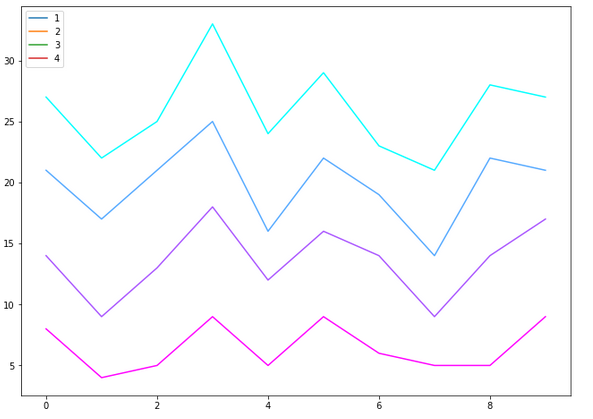### Example 6: Filled Stacked Line Chart

This last example of the line chart tutorial shows us how we can plot a filled stacked line chart. For this plot, we will use fill_between function of matplotlib. This function takes the x-axis and y-axis data, we also pass facecolor parameter for specifying the color to be filled between the lines.

The data passed to fill_between for x-axis and y-axis is generated by using arange and cumsum function of numpy. Here it is shown how you can save the figure plotted into your local system. The savefig helps us in that.

In :
fnx = lambda : np.random.randint(3, 10, 10)
y = np.row_stack((fnx(), fnx(), fnx(), fnx(), fnx()))

x = np.arange(10)
y_stack = np.cumsum(y, axis=0)

fig = plt.figure(figsize=(11,8))

ax1.fill_between(x, 0, y_stack[0,:], facecolor="#CC6666", alpha=0.7)
ax1.fill_between(x, y_stack[0,:], y_stack[1,:], facecolor="#FFACD6", alpha=0.7)
ax1.fill_between(x, y_stack[1,:], y_stack[2,:], facecolor="#6EFF60", alpha=0.7)
ax1.fill_between(x, y_stack[2,:], y_stack[3,:], facecolor="#CC66FF", alpha=0.7)
ax1.fill_between(x, y_stack[3,:], y_stack[4,:], facecolor="#1DFFD6", alpha=0.7)

plt.savefig('final_plot.png')

Output: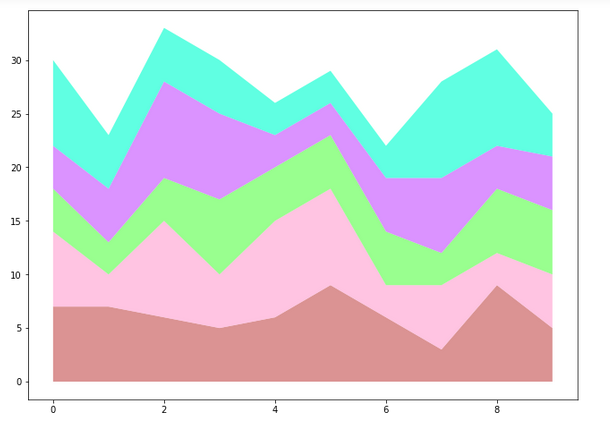## Conclusion

This matplotlib line plot tutorial talked about how you can build a variety of line charts with the help of matplotlib. We learned how different matplotlib functions are used along with numpy functions for plotting useful line plots. Furthermore, we also looked at how we can put markers in our line charts. Along with other line plots, this tutorial also tells about the details that must be taken care while building line charts.

Reference –  Matplotlib Documentation